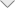### Computer Simulation Software

> > > > Calculation Techniques

### Calculation Techniques

Calculation Techniques

• Fast Direct Solution (FDS) accelerated MoM solver
• Fast Iterative Solution (FIS) based on ACA compression technique and iterative solver
• Green function method for simulation of metallic structures printed on dielectric substrates
• Adaptive Frequency Sampling (AFS) for effective calculations in FD
• Fast Field calculation algorithm
• Matrix Partitioning and Multi Excitation options for extremely fast and accurate optimization calculations
• S, Z, Y matrix calculations for multiport systems
• Physical Optics (PO) method for very high frequencies
• Analysis of mixed electromagnetic and circuit problems
• Recalculate and continue options
• Out-of-core solution for large problems

Parallel Calculations

• Frequency distribution
• Parallel cores for fullwave EM and Hybrid MoM/MTL calculations
• Optimized calculations for small problems
﻿